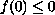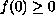Electron. J. Diff. Eqns., Vol. 2001(2001), No. 64, pp. 1-8.

### Stability properties of positive solutions to partial differential equations with delay Gyula Farkas & Peter L. Simon

Abstract:
We investigate the stability of positive stationary solutions of semilinear initial-boundary value problems with delay and convex or concave nonlinearity. If the nonlinearity is monotone, then in the convex caseimplies instability and in the concave caseimplies stability. Special cases are shown where the monotonicity assumption can be weakened or omitted.

Submitted June 21, 2001. Published October 8, 2001.
Math Subject Classifications: 35R10, 35B99.
Key Words: semilinear equations with delay, stability of stationary solutions, convex nonlinearity, concave nonlineariry.

Show me the PDF file (212K), TEX file, and other files for this article.Gyula Farkas Department of Mathematics Istvan Szechenyi College H-9026 Gyor, Hedervariu. 3., Hungary P{rof. Gyula Farkas died in a traffic accident in Lisbon on February 27, 2002. Peter L. Simon School of Chemistry University of Leeds Leeds LS2 9JT, UK e-mail: peters@chem.leeds.ac.uk